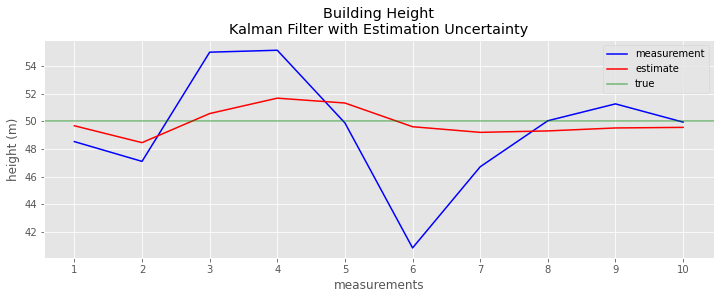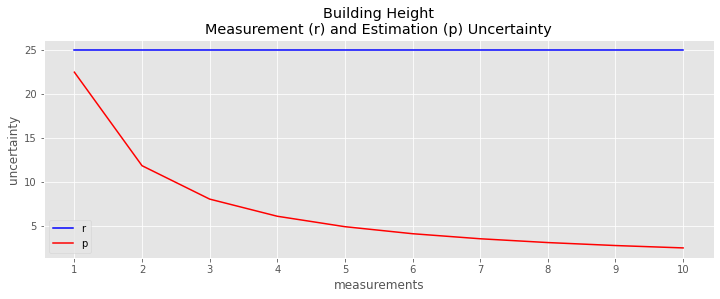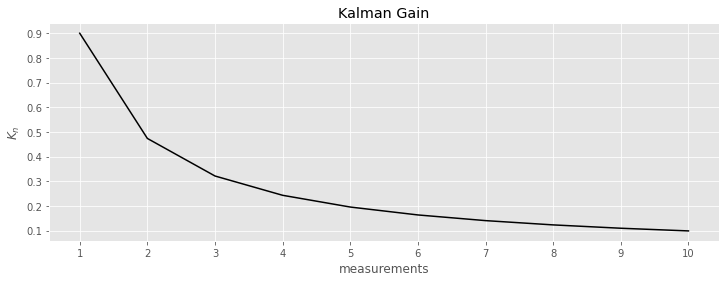# 15. Kalman Filter, II

This notebook is a continuation of on KalmanFilter.NET [Bec18]. In this notebook, we focus on the Kalman Filter in one dimension.

## 15.1. Kalman Gain Equation

Before, the Kalman Gain $$K_n$$ was held fixed or reduced over time by $$\dfrac{1}{n}$$. Here $$K_n$$ changes dynamically and depends on the meaurement device. To start, the Kalman Gain Equation $$K_n$$ is defined as follows.

$$K_n = \dfrac{p_{n,n-1}}{p_{n,n-1} + r_n}$$,

where

• $$p_{n,n-1}$$ is the estimation uncertainty (variance)

• $$r_n$$ is the measurement uncertainty (variance)

• $$0 \leq K_n \leq 1$$

The State Update Equation can be rewritten as follows.

$$\begin{array} \hat{x}_{n,n} & = & \hat{x}_{n,n-1} + K_n (z_n - \hat{x}_{n,n-1}) \\ & = & \hat{x}_{n,n-1} + K_n z_n - K_n \hat{x}_{n,n-1} \\ & = & \hat{x}_{n,n-1} - K_n \hat{x}_{n,n-1} + K_n z_n \\ & = & (1 - K_n) \hat{x}_{n,n-1} + K_n z_n\end{array}$$

When the State Update Equation is written as above, then

• $$(1 - K_n)$$ is the weight given to the estimate

• $$K_n$$ is the weight given to the measurement

The Covariance Update Equation is given as follows.

$$p_{n,n} = (1 - K_n) p_{n,n-1}$$

The Covariance Extrapolation Equations for the position $$x$$ and velocity $$v$$ are give as follows.

• $$p^x_{n+1,n} = p^x_{n,n} + \Delta t^2 p^v_{n,n}$$

• $$p^v_{n+1,n} = p^v_{n,n}$$

## 15.2. Building height, estimation and measurement uncertainties

:

import pandas as pd

data = []

r = 25      # measurement uncertainty
x_ii = 60   # estimate
p_ii = 225  # estimate uncertainty

x_ji = x_ii # prediction
p_ji = p_ii # prediction uncertainty

Z = [48.54, 47.11, 55.01, 55.15, 49.89, 40.85, 46.72, 50.05, 51.27, 49.95]

for z_i in Z:
K_i = p_ji / (p_ji + r)

x_ii = x_ji + K_i * (z_i - x_ji)
p_ii = (1 - K_i) * p_ji

x_ji = x_ii
p_ji = p_ii

data.append({
'K_i': K_i,
'x_ii': x_ii,
'p_ii': p_ii,
'z_i': z_i,
'r_i': r
})

data = pd.DataFrame(data)
data.index = range(1, data.shape + 1)
data

:

K_i x_ii p_ii z_i r_i
1 0.900000 49.686000 22.500000 48.54 25
2 0.473684 48.465789 11.842105 47.11 25
3 0.321429 50.569286 8.035714 55.01 25
4 0.243243 51.683514 6.081081 55.15 25
5 0.195652 51.332609 4.891304 49.89 25
6 0.163636 49.617273 4.090909 40.85 25
7 0.140625 49.209844 3.515625 46.72 25
8 0.123288 49.313425 3.082192 50.05 25
9 0.109756 49.528171 2.743902 51.27 25
10 0.098901 49.569890 2.472527 49.95 25
:

import matplotlib.pyplot as plt

plt.style.use('ggplot')

fig, ax = plt.subplots(figsize=(12, 4))

_ = data.z_i.plot(kind='line', ax=ax, color='blue', label='measurement')
_ = data.x_ii.plot(kind='line', ax=ax, color='red', label='estimate')
_ = ax.axhline(50, color='green', alpha=0.5, label='true')
_ = ax.set_xticks(range(1, data.shape + 1))
_ = ax.set_xticklabels(data.index)
_ = ax.legend()
_ = ax.set_xlabel('measurements')
_ = ax.set_ylabel('height (m)')
_ = ax.set_title('Building Height\nKalman Filter with Estimation Uncertainty'):

fig, ax = plt.subplots(figsize=(12, 4))

_ = data.r_i.plot(kind='line', ax=ax, color='blue', label='r')
_ = data.p_ii.plot(kind='line', ax=ax, color='red', label='p')
_ = ax.set_xticks(range(1, data.shape + 1))
_ = ax.set_xticklabels(data.index)
_ = ax.legend()
_ = ax.set_xlabel('measurements')
_ = ax.set_ylabel('uncertainty')
_ = ax.set_title('Building Height\nMeasurement (r) and Estimation (p) Uncertainty')[ ]:

fig, ax = plt.subplots(figsize=(12, 4))

_ = data.K_i.plot(kind='line', ax=ax, color='black')
_ = ax.set_xticks(range(1, data.shape + 1))
_ = ax.set_xticklabels(data.index)
_ = ax.set_xlabel('measurements')
_ = ax.set_ylabel(r'$K_n$')
_ = ax.set_title('Kalman Gain')